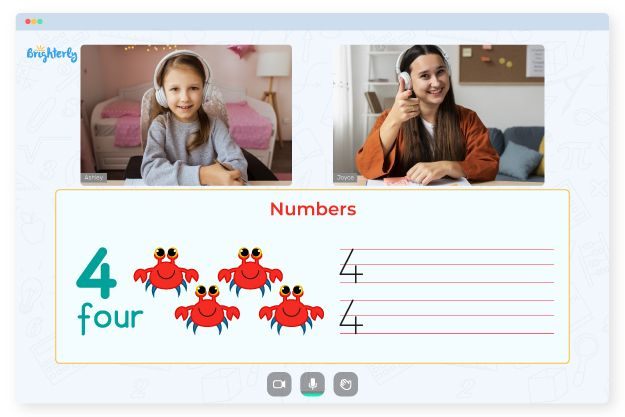# Doubles Facts Worksheet

Elementary school students must remember double facts to progress in their math education. Mastering double facts can benefit students’ number sense, mental math skills, and overall math confidence. Therefore, teachers frequently provide worksheets that concentrate specifically on these kinds of additional problems to assist students in practicing their double facts.

## Double Facts Worksheets

Basic addition facts involving adding a number are known as doubles facts. Because they serve as the foundation for more advanced concepts in addition and subtraction, younger students need to have a firm grasp of these facts. Teachers can reinforce these ideas using various teaching resources, including doubles math fact worksheets.

Math for Kids

Is Your Child Struggling With Math?
1:1 Online Math TutoringA valuable resource for teaching students to memorize and master double facts is a worksheet. Most of the time, the worksheet has a series of addition problems involving double facts. Equations, number sentences, and word problems are just a few formats tutors can use to present these challenges.

## Benefits

Double facts worksheets are highly beneficial for young learners to develop fluency and conceptual understanding in essential addition. As a result, the use of a doubles math facts worksheet is an excellent way for young learners to strengthen their mathematical foundation and pave the way for future academic success.

Students can build a solid foundation for more complex addition and subtraction concepts with repeated practice of simple facts using these educational tools. Consequently, it is crucial to develop their computational abilities and mathematical self-assurance.### Doubles Facts Worksheet PDF

Double Facts Worksheets### Doubles Facts Worksheet PDF

Doubles Facts Worksheet### Doubles Facts Worksheet PDF

Doubles Math Fact Worksheets### Doubles Facts Worksheet PDF

Doubles Math Facts Worksheet

Struggling with Numbers?• Is your child having trouble understanding the fundamentals of numbers?
• Try studying with an online tutor.

Does your child need extra help with the concept of numbers? Start lessons with an online tutor.